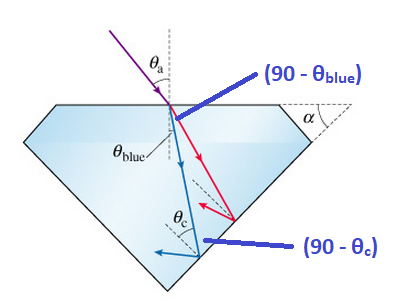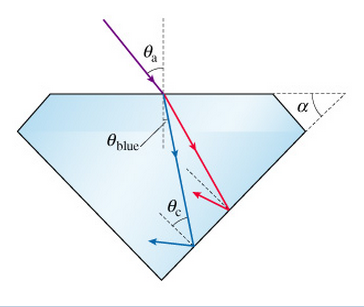# Problem: A beam of white light is incident on the surface of a diamond at an angle θa. (Figure 1) Since the index of refraction depends on the light's wavelength, the different colors that comprise white light will spread out as they pass through the diamond. For example, the indices of refraction in diamond are nred = 2.410 for red light and nblue = 2.450 for blue light. Thus, blue light and red light are refracted at different angles inside the diamond, as shown in the picture. The surrounding air has nair = 1.000. Note that the angles in the figure are not to scale.A diamond is cut such that the angle between its top surface and its bottom surface is α. For α = 45°, find the largest possible value of the incident angle θa such that the blue light is totally internally reflected off the bottom surface.

###### FREE Expert Solution

Snell's law:

$\overline{){{\mathbf{\eta }}}_{{\mathbf{1}}}{\mathbf{s}}{\mathbf{i}}{\mathbf{n}}{{\mathbf{\theta }}}_{{\mathbf{1}}}{\mathbf{=}}{{\mathbf{\eta }}}_{{\mathbf{2}}}{\mathbf{s}}{\mathbf{i}}{\mathbf{n}}{{\mathbf{\theta }}}_{{\mathbf{2}}}}$

Critical angle:

$\overline{){{\mathbf{\theta }}}_{{\mathbf{c}}}{\mathbf{=}}{\mathbf{s}}{\mathbf{i}}{{\mathbf{n}}}^{\mathbf{-}\mathbf{1}}{\mathbf{\left(}}\frac{{\mathbf{\eta }}_{\mathbf{2}}}{{\mathbf{\eta }}_{\mathbf{1}}}{\mathbf{\right)}}}$η1 = ηblue = 2.450

η2 = ηair = 1

θc = sin-1airblue) = sin-1 (1/2.4500) = 24.09°

From the sum of angles in a triangle:###### Problem Details

A beam of white light is incident on the surface of a diamond at an angle θa. (Figure 1) Since the index of refraction depends on the light's wavelength, the different colors that comprise white light will spread out as they pass through the diamond. For example, the indices of refraction in diamond are nred = 2.410 for red light and nblue = 2.450 for blue light. Thus, blue light and red light are refracted at different angles inside the diamond, as shown in the picture. The surrounding air has nair = 1.000. Note that the angles in the figure are not to scale.

A diamond is cut such that the angle between its top surface and its bottom surface is α. For α = 45°, find the largest possible value of the incident angle θa such that the blue light is totally internally reflected off the bottom surface.